# Forces on earth directions

A force of 60 N [North] and 80 N [East] is exerted on an object wigth 10 kg. What is the acceleration of the object?

a =  10 m/s2

### Step-by-step explanation: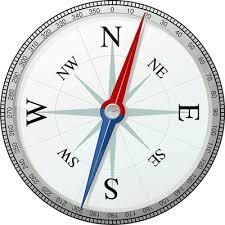Did you find an error or inaccuracy? Feel free to write us. Thank you!Tips to related online calculators
Our vector sum calculator can add two vectors given by their magnitudes and by included angle.
Pythagorean theorem is the base for the right triangle calculator.

#### You need to know the following knowledge to solve this word math problem:

We encourage you to watch this tutorial video on this math problem:

## Related math problems and questions:

• Forces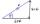Determine the resultant of two perpendicular forces F1 = 560 N and second force of 25% smaller.
• Acceleration 2if a car traveling at a velocity of 80 m/s/south accelerated to a velocity of 100 m/s east in 5 seconds, what is the cars acceleration? using Pythagorean theorem
• Two forcesTwo forces with magnitudes of 25 and 30 pounds act on an object at 10° and 100° angles. Find the direction and magnitude of the resultant force. Round to two decimal places in all intermediate steps and your final answer.
• Chained winch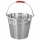When digging a well, a chained winch is used to balance the material. An empty shackle weighs 8 kg, a full 64 kg. What force must the worker exert on the crank handle when pulling the shackle with a uniform movement from a depth of 5 m if the shaft diamet
• Two boys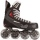Two boys weighing 60kg and 90kg move on roller skates and are attracted to each other by a rope. One boy exerts a force of 15N. What force does the other boy exert? What accelerations will the boys gain? Do not consider friction or air resistance.
• A stone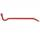There is a stone weighing 60 kg on the billet. The distance from the support point to the stone is 20cm. The length of the billet is 1m. Determine the force exerted by the hand at the end of the billet.
• Center of gravityThe mass points are distributed in space as follows - specify by coordinates and weight. Find the center of gravity of the mass points system: A1 [1; -20; 3] m1 = 46 kg A2 [-20; 2; 9] m2 = 81 kg A3 [9
• Hydrostatic forceWhat is the hydrostatic force applied to an area of 30 cm2 in the water at a depth of 20 m? (Water density is 1000 kg/m3)
• Mass point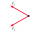Two equal forces of 30 Newtons act on a mass point. Find the magnitude of the resultant force if these forces form an angle of 42°.
• Inclined plane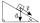1. How much work W we have to do to pull a body weighing 200 kg along an inclined plane with a length of 4 m to a total height of 1.5 m. 2. Find the force we need to exert to do this if we neglect frictional resistance. 3. Find the force we would need if
• The carThe car weight 1280 kg, increased its speed from 7.3 m/s to 63 km/h on a track of 37.2 m. What force did the car engine have to exert?
• The aluminumThe aluminum object with a weight of 0.80 kg and a temperature of 250°C was placed in the water with a weight of 1.6 kg and a temperature of 23°C. What is the system temperature after reaching equilibrium? We assume that the heat exchange occurred only be
• CarAt what horizontal distance reaches the car weight m = 753 kg speed v = 74 km/h when the car engine develops a tensile force F = 3061 N. (Neglect resistance of the environment.)
• Cuboid 5Calculate the mass of the cuboid with dimensions of 12 cm; 0.8 dm and 100 mm made from spruce wood (density = 550 kg/m3).
• Heat transferWe placed a lead object weighing 0.4 kg and 250°C in 0.4 L water. What was the initial temperature of water t2 if the object's temperature and the water after reaching equilibrium was 35°C? We assume that the heat exchange occurred only between the lead o
• Positional energyWhat velocity in km/h must a body weighing 60 kg have for its kinetic energy to be the same as its positional energy at the height 50 m?
• Inclined plane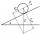On the inclined plane with an inclination angle of 30°, we will put the body (fixed point) with mass 9 kg. Determine the acceleration of the body motion on an inclined plane.